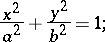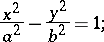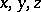# Cylindrical surface (cylinder)

(diff) ← Older revision | Latest revision (diff) | Newer revision → (diff)

The surface formed by the motion of a line (the generator) moving parallel to itself and intersecting a given curve (the directrix).

The directrix of a cylindrical surface of the second order is a curve of the second order. Depending on the form of the directrix one distinguishes an elliptic cylinder, the canonical equation of which isan imaginary elliptic cylinder:and a parabolic cylinder:If the directrix is a degenerate curve of the second order (i.e. a pair of lines), then the cylindrical surface is a pair of planes (intersecting, parallel or coincident, real or imaginary, depending on the corresponding property of the directrix).

A cylindrical surface of orderis an algebraic surface given in some affine coordinate systemby an algebraic equation of degreenot containing one of the coordinates (for example,):(*)

The curve of orderdefined by equation (*) is sometimes called the base of the cylindrical surface.

How to Cite This Entry:
Cylindrical surface (cylinder). Encyclopedia of Mathematics. URL: http://encyclopediaofmath.org/index.php?title=Cylindrical_surface_(cylinder)&oldid=15188
This article was adapted from an original article by A.B. Ivanov (originator), which appeared in Encyclopedia of Mathematics - ISBN 1402006098. See original article